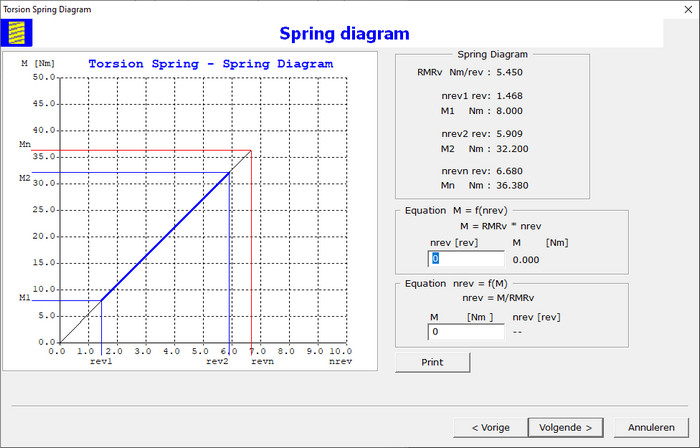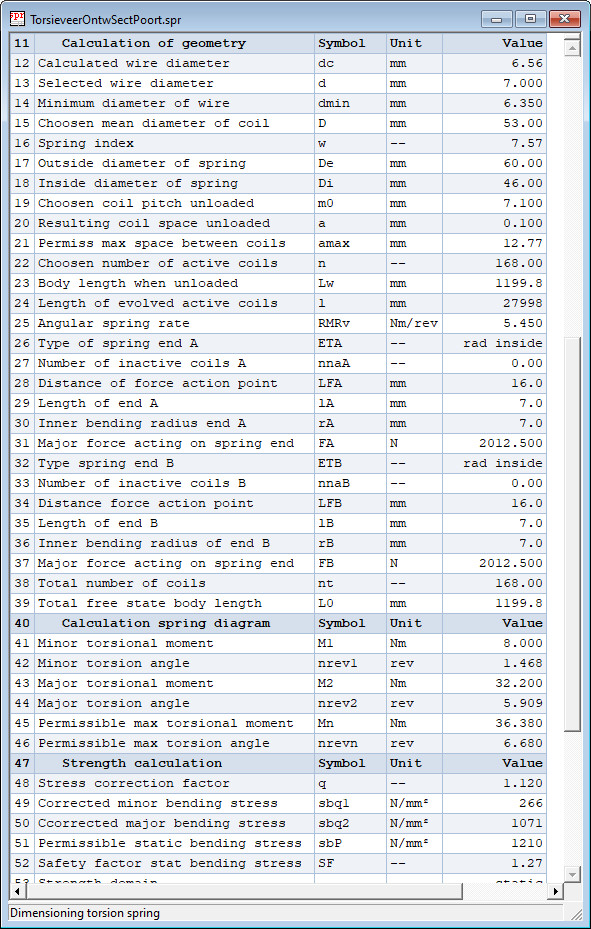### Spring Diagram

Spring diagram is displayed in wizard page:The equation of spring line M = RMR * α or M = RMRv * nrev is determined. Get with this equation the torsional moment M for a given angle α or number of revolutions nrev. With the inverse equation α = M/RMR or nrev = M/RMRv the angle α or number of revolutions nrev is determined to obtain a given torsional moment M. The maximum permissible torsion angle αn or torsional revolutions nrevn and maximum permissible torsional moment Mn are calculated.

### Output Calculation Design Torsion Spring

The calculation results are displayed in table form on the screen, as in following example:Click here on Calculation Report Design Torsion Spring to see a complete calculation report for dessign of a torsion spring in a target blank page.

## Verification Calculation of a Torsion Spring

The verification calculation can only be done if the dimensions of the spring and the load are known. The calculation is done according the standard DIN EN 13906-3. The safety factors for static and dynamic failure are calcualted. The same calculation steps as in the design operation are performed, except those for calculation of the dimensions. The natural frequency in transverse direction is calculated.

Click here on Calculation Report Verification Torsion Spring to see a complete calculation report of a verification of torsion spring in a target blank page.

End of web pages HeliSpring. Go to the Products List to read about another product.